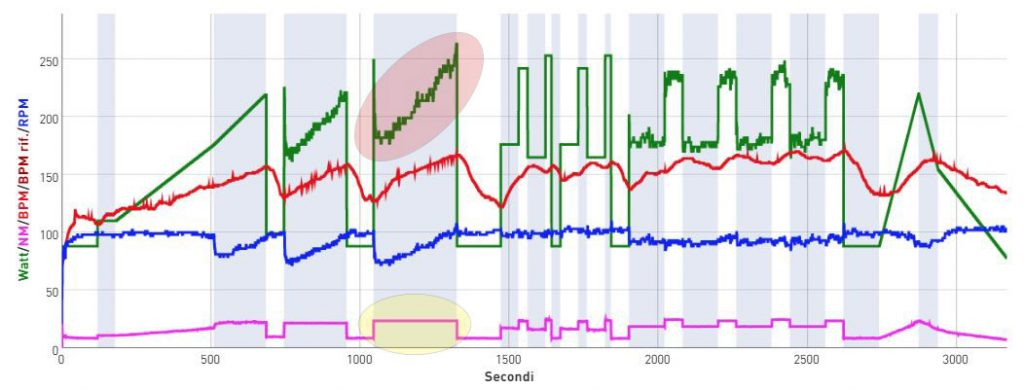# Cycling Progression at fixed value of Newton

The MagneticDays Head Coach Simone Buracchi introduces another one fundamental cycling exercise of HTT Methodology of Training: THE CYCLING PROGRESSION AT FIXED VALUE OF NEWTON (WITH INCREASE OF WATT + RPM).

“Cadence increases as force (load applied to your pedals) increases, under indoor and outdoor conditions. In this case (as shown in the graph below), pedalling frequency and Watts increase (rec circle) but not the force applied to your pedals (Newton) that remains unvaried (fixed value of Newton at the threshold as shown in the yellow circle). What do we mean when we talk about Cycling Progression at fixed value of Newton? The purpose of this type of cycling exercise (that can only be done with JARVIS smart trainer) is to work separately on Force and Cadence in a focused way. Generally (outdoor conditions), cadence increases (with the same bike gear ratio) of 5 Watts for 1 pedalling that are equally distributed between Newton and RPM. In this case – as shown in the graph below – the value of Newton is fixed and Watts increase of 3 for 1 pedalling. So, the cardio-respiratory system works more than the muscular one if necessary to rebalance these two components.”